### GATE 2014 ECE Video Solution on Digital Circuits (Digital Electronics)

Set – 1 (15th February 2014 (Forenoon))
1. In the following circuit employing pass transistor logic, all NMOS transistors are identical with a threshold voltage of 1 volt. Ignoring the body effect, the output voltages at P, Q, and R are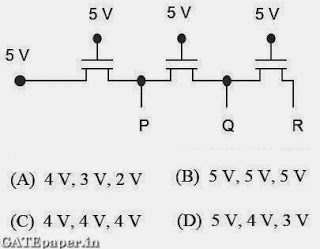2. The Boolean expression simplifies to3. Five JK flip flops are cascaded to form the circuit shown in figure. Clock pulses at a frequency of 1 MHz are applied as shown. The frequency (in KHz) of the waveform at Q3 is …………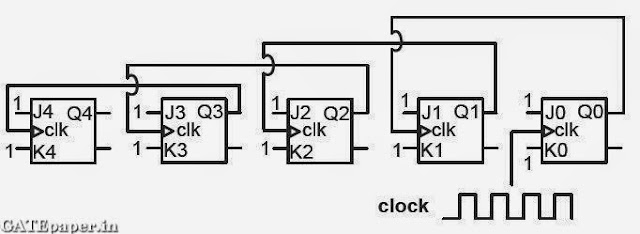4. The output F in the digital logic circuit shown in the figure is5. For the given Boolean function, which one of the following is the complete set of essential prime implicants?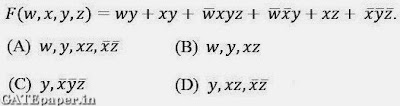6. The digital logic shown in the figure satisfies the given state diagram when Q1 is connected to input A of the XOR gate. Suppose the XOR gate is replaced by the XNOR gate. Which one of the following options preserves the state diagram?SET – 2 (15th February 2014 (Afternoon))
1. For an n – variable Boolean function, the maximum number of prime implicants is
a. 2(n – 1)
b. n/2
c. 2n
d. 2(n – 1)

2. The number of bytes required to represent the decimal number 1856357 in packed BCD (Binary Coded Decimal) form is ………….

3. In a half-subtractor circuit with X and Y as inputs, the Borrow (M) and Difference (N = X – Y) are given by4. In the circuit shown, choose the correct timing diagram of the output (y) from the given waveforms W1, W2, W3 and W4.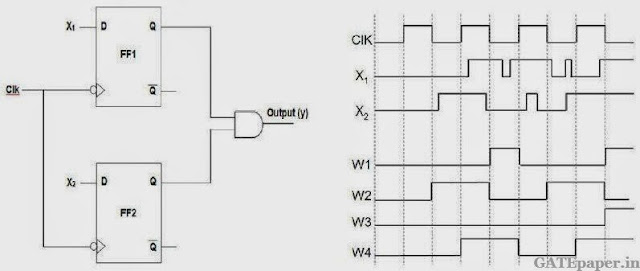a. W1
b. W2
c. W3
d. W4

5. The outputs of the two flip-flops Q1, Q2 in the figure shown are initialized to 0, 0. The sequence generated at Q1 upon application of clock signal is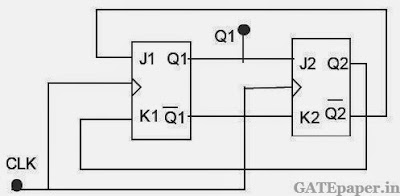a. 01110….
b. 01010…..
c. 00110….
d. 01100…..

6. For the 8085 microprocessor, the interfacing circuit to input 8 – bit digital data (DI0 – DI7) from an external device is shown in figure. The instruction for correct data transfer isa. MVI A, F8H
b. IN F8H
c. OUT F8H
d. LDA F8F8H

SET – 3 (16th February 2014 (Forenoon))
1. An analog voltage in the range 0 to 8 volts is divided in 16 equal intervals for conversion to 4 – bit digital output. The maximum quantization error (in volts) is ………..

2. The circuit shown in the figure is aa. Toggle flip flop
b. JK flip flop
c. SR Latch
d. Master – Slave D flip flop

3. Consider the multiplexer based logic circuit shown in the figure.4. If WL is the Word Line and BL is the Bit Line, an SRAM cell is shown is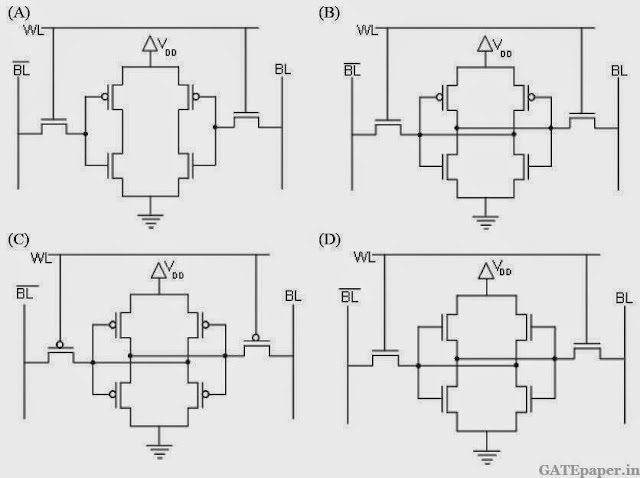5. In the circuit shown, W and Y are MSBs of the control inputs. The output F is given by6. If X and Y are inputs and the Difference (D = X – Y) and the Borrow (B) are the outputs, which one of the following diagrams implements a half-subtractor?SET - 4 (16th February 2014 (Afternoon))

1. For a given sample and hold circuit, if the value of the hold capacitor is increased, then
a. Droop rate decreases and Acquisition time decreases
b. Droop rate decreases and Acquisition time increases
c. Droop rate increases and Acquisition time decreases
d. Droop rate increases and Acquisition time increases

2. In the circuit shown in the figure, if C = 0, the expression for Y is3. The output (Y) of the circuit shown in the figure is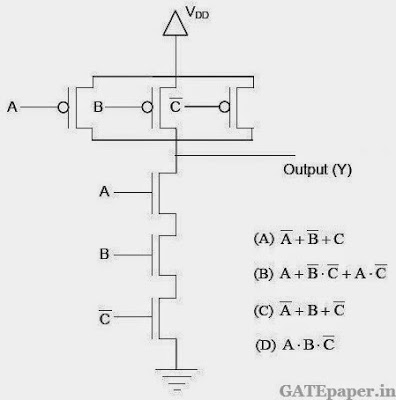4. An 8 – to – 1 multiplexer is used to implement a logical function Y as shown in the figure. The output Y is given by5. A 16 bit ripple carry adder is realized using 16 identical full adders (FA) as shown in the figure. The carry propagation delay of each FA is 12 ns and the sum propagation delay of each FA is 15 ns. The worst case delay (in ns) of this 16 – bit adder will be…………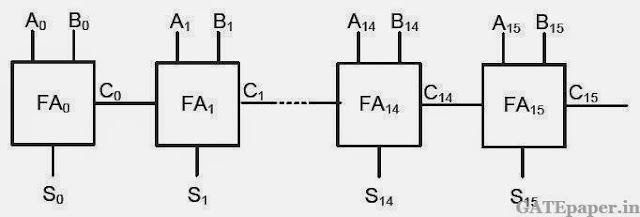6. An 8085 microprocessor executes “STA 1234H” with starting address location 1FFEH (STA copies the contents of the accumulator to the 16 bit address location). While the instruction is fetched and executed, the sequence of values written at the address pins A15 - A8 is